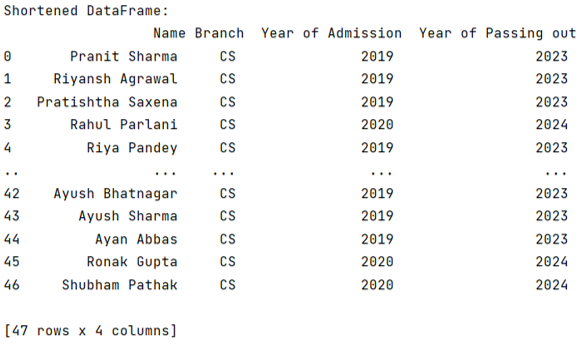# How to set number of maximum rows in Pandas DataFrame?

Given a Pandas DataFrame, we have to set the number of maximum rows. By Pranit Sharma Last updated : September 21, 2023

In the real world, data is huge so is the dataset. While importing a dataset and converting it into DataFrame, if the number of columns or rows is large, the default printing method does not print the entire columns. It compresses the rows and columns.

## Problem statement

Given a Pandas DataFrame, we have to set the number of maximum rows.

## Setting number of maximum rows in Pandas DataFrame

In case we need to maximize the number of rows in a pandas DataFrame, we will use pd.set_option('display.max_rows', n), where n is the maximum number of rows we want to display.

### Step 1: Import pandas package

To work with pandas, we need to import pandas package first, below is the syntax:

```import pandas as pd
```

Let us understand with the help of an example.

### Step 2: Create and print pandas dataframe

```# Importing pandas package
import pandas as pd

# Importing a csv file having
# large number of files

# Creating a DataFrame
df = pd.DataFrame(d)

# Display original DataFrame
print("Original DataFrame:\n",df,"\n")
```

The output of the above program is:### Step 3: Set number of maximum rows and print them

Here, we are having a DataFrame having 47 number of rows, suppose we want don't want all the rows to be printed and only 10 rows to save our time, we will use pd.set_option('display.max_rows', 10).

```# Setting max number of rows
pd.set_option('display.max_rows', 10)

# Display Shortened DataFrame
print("Shortened DataFrame:\n",df,"\n")
```

The output of the above program is: